# `sklearn.model_selection`.train_test_split¶

`sklearn.model_selection.``train_test_split`(*arrays, **options)[source]

Split arrays or matrices into random train and test subsets

Quick utility that wraps input validation and `next(ShuffleSplit().split(X, y))` and application to input data into a single call for splitting (and optionally subsampling) data in a oneliner.

Read more in the User Guide.

Parameters: *arrays : sequence of indexables with same length / shape Allowed inputs are lists, numpy arrays, scipy-sparse matrices or pandas dataframes. test_size : float, int, or None (default is None) If float, should be between 0.0 and 1.0 and represent the proportion of the dataset to include in the test split. If int, represents the absolute number of test samples. If None, the value is automatically set to the complement of the train size. If train size is also None, test size is set to 0.25. train_size : float, int, or None (default is None) If float, should be between 0.0 and 1.0 and represent the proportion of the dataset to include in the train split. If int, represents the absolute number of train samples. If None, the value is automatically set to the complement of the test size. random_state : int or RandomState Pseudo-random number generator state used for random sampling. stratify : array-like or None (default is None) If not None, data is split in a stratified fashion, using this as the class labels. splitting : list, length=2 * len(arrays) List containing train-test split of inputs. New in version 0.16: If the input is sparse, the output will be a `scipy.sparse.csr_matrix`. Else, output type is the same as the input type.

Examples

```>>> import numpy as np
>>> from sklearn.model_selection import train_test_split
>>> X, y = np.arange(10).reshape((5, 2)), range(5)
>>> X
array([[0, 1],
[2, 3],
[4, 5],
[6, 7],
[8, 9]])
>>> list(y)
[0, 1, 2, 3, 4]
```
```>>> X_train, X_test, y_train, y_test = train_test_split(
...     X, y, test_size=0.33, random_state=42)
...
>>> X_train
array([[4, 5],
[0, 1],
[6, 7]])
>>> y_train
[2, 0, 3]
>>> X_test
array([[2, 3],
[8, 9]])
>>> y_test
[1, 4]
```

## Examples using `sklearn.model_selection.train_test_split`¶Faces recognition example using eigenfaces and SVMs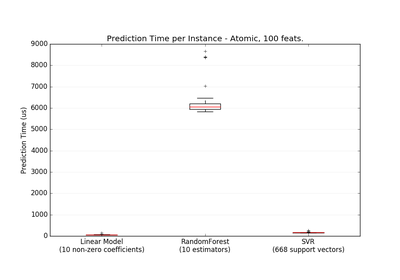Prediction Latency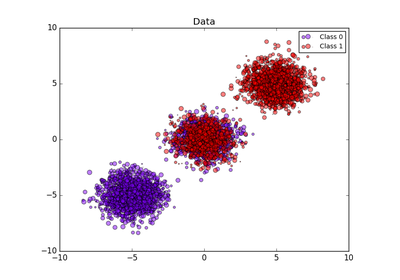Probability calibration of classifiers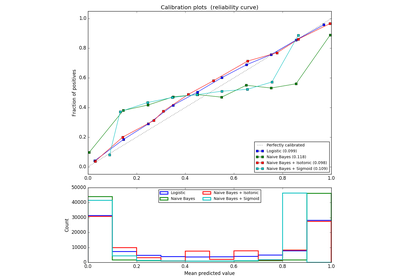Probability Calibration curves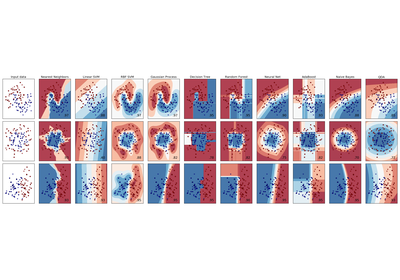Classifier comparison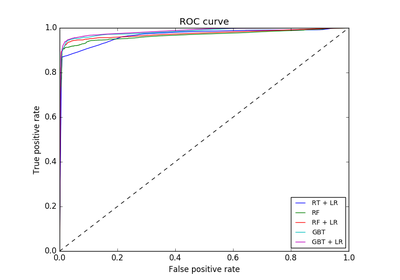Feature transformations with ensembles of trees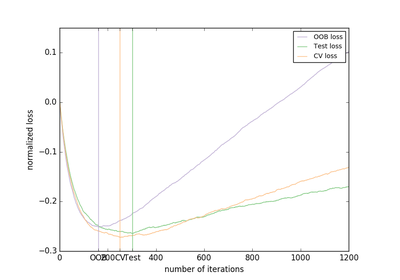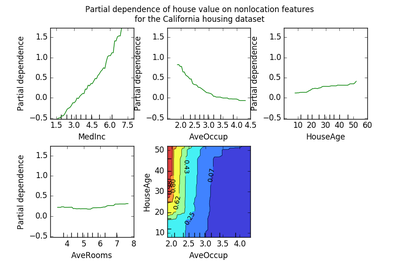Partial Dependence Plots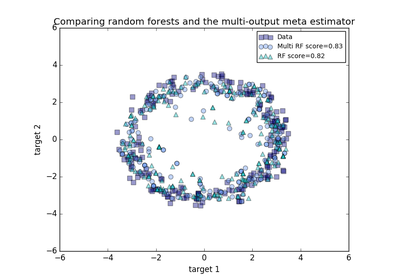Comparing random forests and the multi-output meta estimator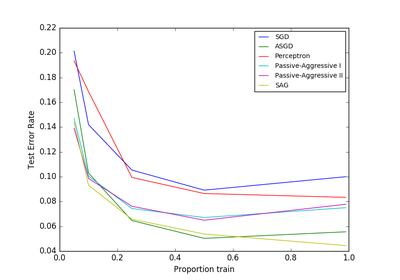Comparing various online solversParameter estimation using grid search with cross-validation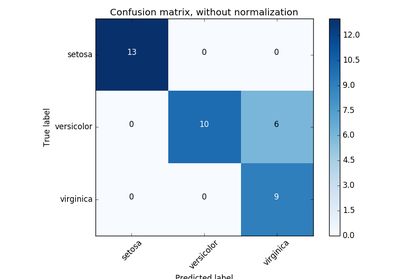Confusion matrix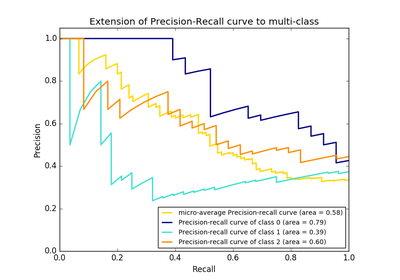Precision-Recall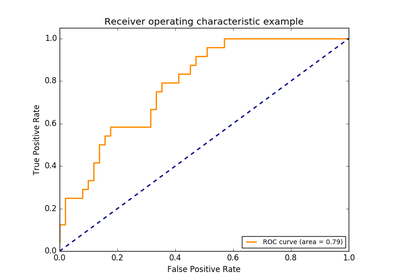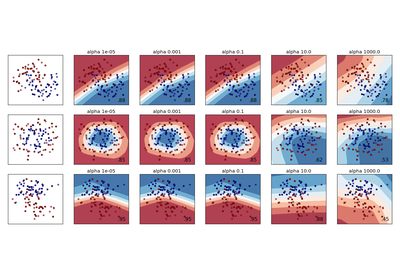Varying regularization in Multi-layer Perceptron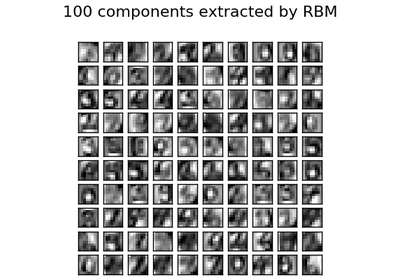Restricted Boltzmann Machine features for digit classification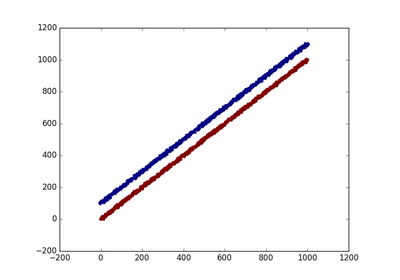Using FunctionTransformer to select columnsUnderstanding the decision tree structure